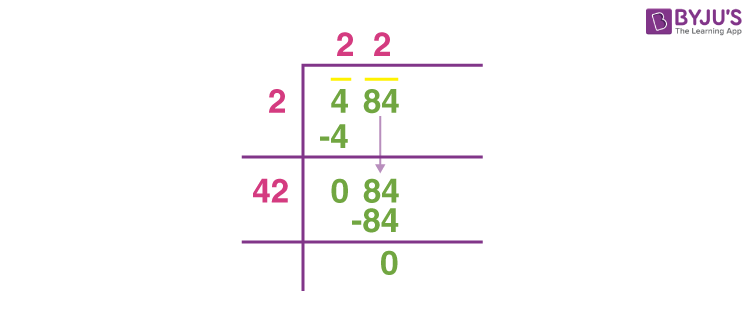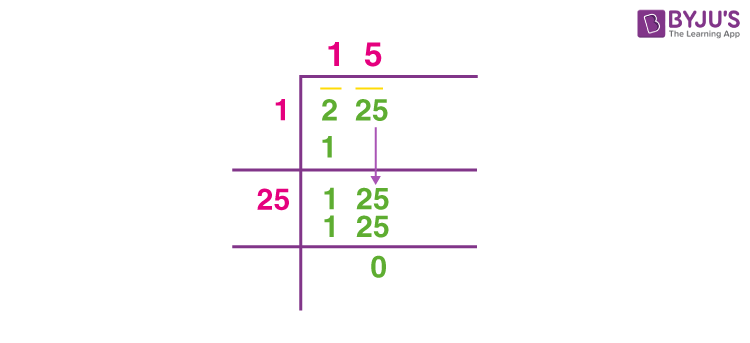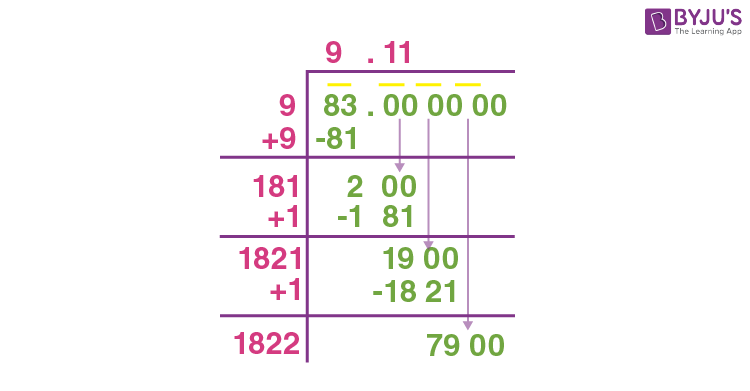# Finding Square Root Of A Number By Division Method

Square root by long division method: Any number can be expressed as a product of prime numbers. This method of representation of a number in terms of the product of prime numbers is termed as the prime factorization method. It is the easiest method known for the manual calculation of the square root of a number. But this method becomes tedious and tiresome when the number involved is large. Therefore, to find the square root of large numbers we use the long division method.

Also, to find the square roots of imperfect squares such as 2, 3, 5, 6, 8, etc., we can use a long division method avoiding the use of calculators. As we know the division method is used to find the square root of a number, prime factors of a given number, lcm and HCF of given numbers. In this article, you will learn how to solve square root problems using a division method.

The division method of the square root is a very familiar and easy method available to get the accurate roots of numbers. In this method, we can see 5 major steps such as divide, multiply, subtract, bring down and repeat.

## Square root of a number by long division method

Let us understand the long division method with the help of an example.

1. Taking 484 as the number whose square root is to be evaluated. Place a bar over the pair of numbers starting from the unit place or Right-hand side of the number. In case, if we have the total number of digits as odd number, the leftmost digit will also have a bar,
$$\begin{array}{l}i.e.,\ \bar{4} \ and\ \bar{84}\end{array}$$
2. Take the largest number as the divisor whose square is less than or equal to the number on the extreme left of the number. The digit on the extreme left is the dividend. Divide and write the quotient. Here the quotient is 2 and the remainder is 0.3. Next, we then bring down the number, which is under the bar, to the right side of the remainder. Here, in this case, we bring down 84. Now, 84 is our new dividend.4. Now double the value of the quotient and enter it with a blank space on the right side.5. Next, we have to select the largest digit for the unit place of the divisor (4_) such that the new number, when multiplied by the new digit at the unit’s place, is equal to or less than the dividend (84).

In this case,  42 × 2 = 84. So the new digit is 2.1. The remainder is 0, and we have no number left for division, therefore,
$$\begin{array}{l}\sqrt{484} = 22\end{array}$$

## Video Lessons on Square Roots

### Visualising square roots### Finding Square roots### More Square Roots

Check here to find the square root of more numbers such as 2, 3, 5, 7, 8, etc. using a long division method.

## Solved Examples

Example 1:

Find the square root of 225 by the long division method.

Solution:

By long division method, we can get the square root of 225 as:Therefore, √225 = 15.

Example 2:

Calculate the square root of 83.

Solution:

By long division method, we can calculate the square root of 83 as:Hence, √83 = 9.11 (approx)

### Practice Problems

1. Estimate the square root of 441.
2. What is teh square root of 200?
3. Find the value of √20 + √144.

## Frequently Asked Questions – FAQs

Q1

### How do you divide step by step?

Division method can be explained in steps using four operations namely, divide, multiply, subtract and bring down.
Step 1: Divide the given number by divisor by identifying the suitable integer.
Step 2: Multiply the divisor and integer (quotient) to get the number to be subtracted from the dividend.
Step 3: Subtract the number from the dividend.
Step 4: Bring down the remainder and another digit (if any) from the dividend.
Step 5: Continue the above process till the remainder is 0 or less than the divisor.
Q2

### What are the three ways to divide?

We can divide the numbers in three ways using a box, sign and bar. However, the result in all three ways will be the same. These can be expressed as:
Divisor) Dividend ( quotient
Dividend ÷ Divisor = quotient
Dividend/Divisor = quotient
Q3

### How do you divide correctly?

We can divide the given numbers correctly with the help of multiplication tables. As we can get the right quotients when we know the multiples of divisors.
Q4

### What are the 5 steps of long division?

The five steps of long division are given by:
Step 1: Divide
Step 2: Multiply
Step 3: Subtract
Step 4: Bring down
Step 5: Repeat
Q5

### What are symbols for division?

The symbols used for division are ÷, slash (/) or a horizontal line ( _ ).
Test your Knowledge on Square Root Long Division Method